# The switch in the circuit in (Figure 1) has been in the left position for a long time

The switch in the circuit in (Figure 1) has been in the left position for a long time. At t = 0, it moves to the right position and stays there.

Part A

Select the correct expression for the capacitor voltage, v(t), fort t ≥ 0

Part B Select the correct expression for the current through the 24 kΩ resistor i(t) ≥ 0+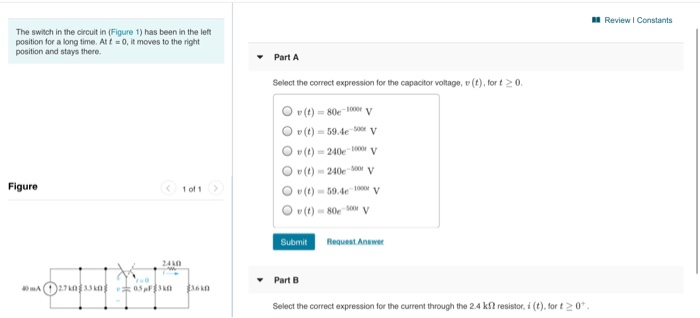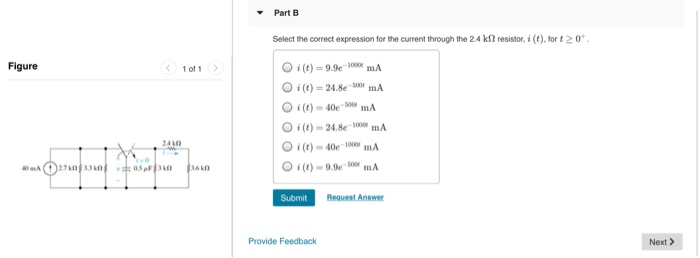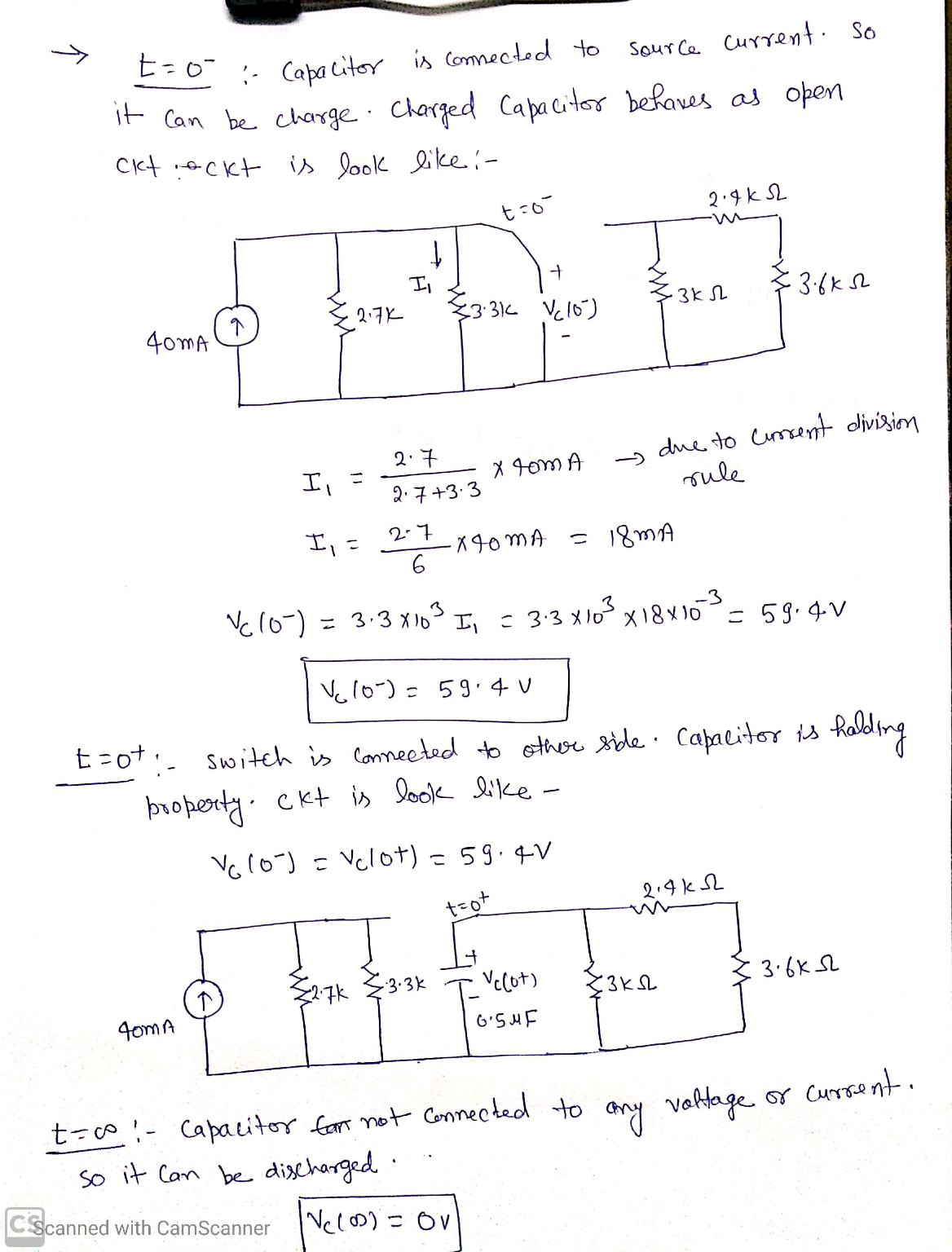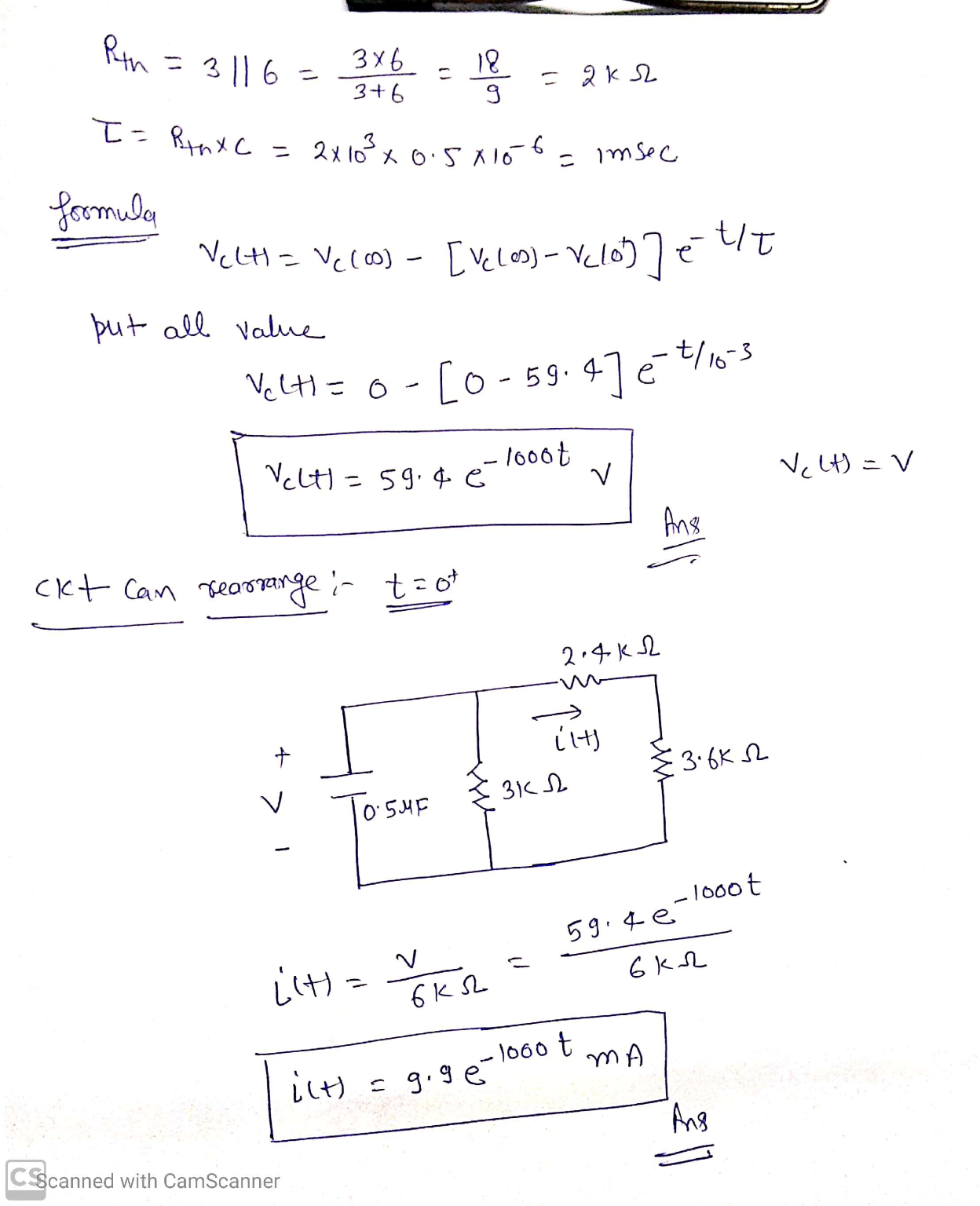#### Earn Coin

Coins can be redeemed for fabulous gifts.

Similar Homework Help Questions
• ### The switch in the circuit in (Figure 1) has been in the left position for a...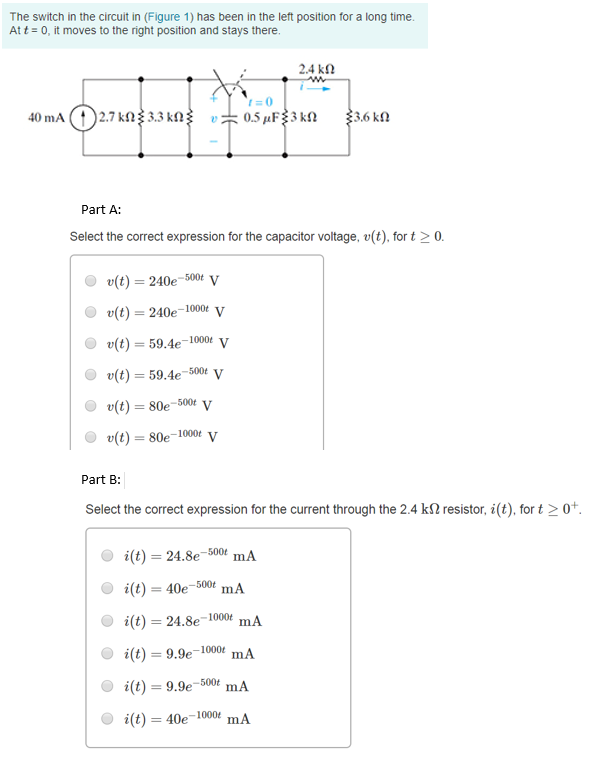The switch in the circuit in (Figure 1) has been in the left position for a long time Att 0, it moves to the right position and stays there 2.4 kΩ Part A Select the correct expression for the capacitor voltage, v(t), for t 0 (t) 240e-500t y (t) 240e-1000 v O r(t)-59.4e-1000e V v(t)59.4e-00 V (t) 80e-50 V (t)-80e-1000t V Part B: Select the correct expression for the current through the 2.4 kΩ resistor, i (t), for t >...

• ### 4. The switch in the circuit below has been in the left positiorn for a long...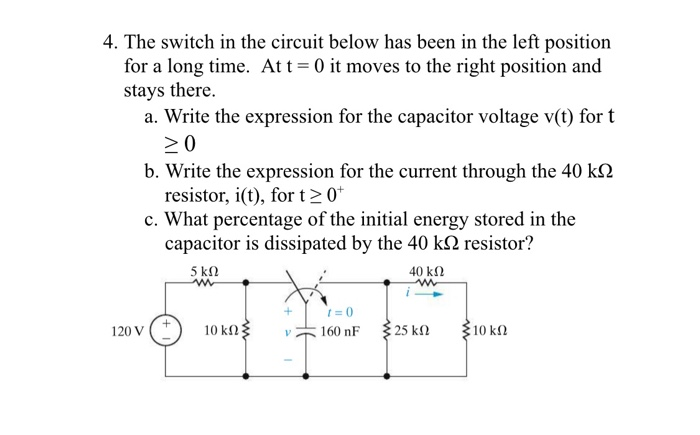4. The switch in the circuit below has been in the left positiorn for a long time. Att -0 it moves to the right position and stays there. a. Write the expression for the capacitor voltage v(t) for t 20 r the current through the 40 kΩ resistor, i(t), for t2 0 c. What percentage of the initial energy stored in the capacitor is dissipated by the 40 kS2 resistor? 200 i

• ### Consider the RC circuit in the figure below. The switch was at position a for a long period of time and it is suddenly...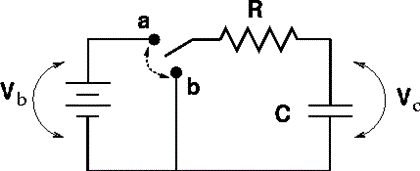Consider the RC circuit in the figure below. The switch was at position a for a long period of time and it is suddenly switched to position b at time t = 0. For each statement select True or False. 1. The current through the resistor equals the current across the capacitor at all times. 2. In the instant after the switch is thrown the current across the capacitor is zero. 3. In the instant after the switch is thrown...

• ### 1. The switch has been in the position shown for a long time. Find the charge...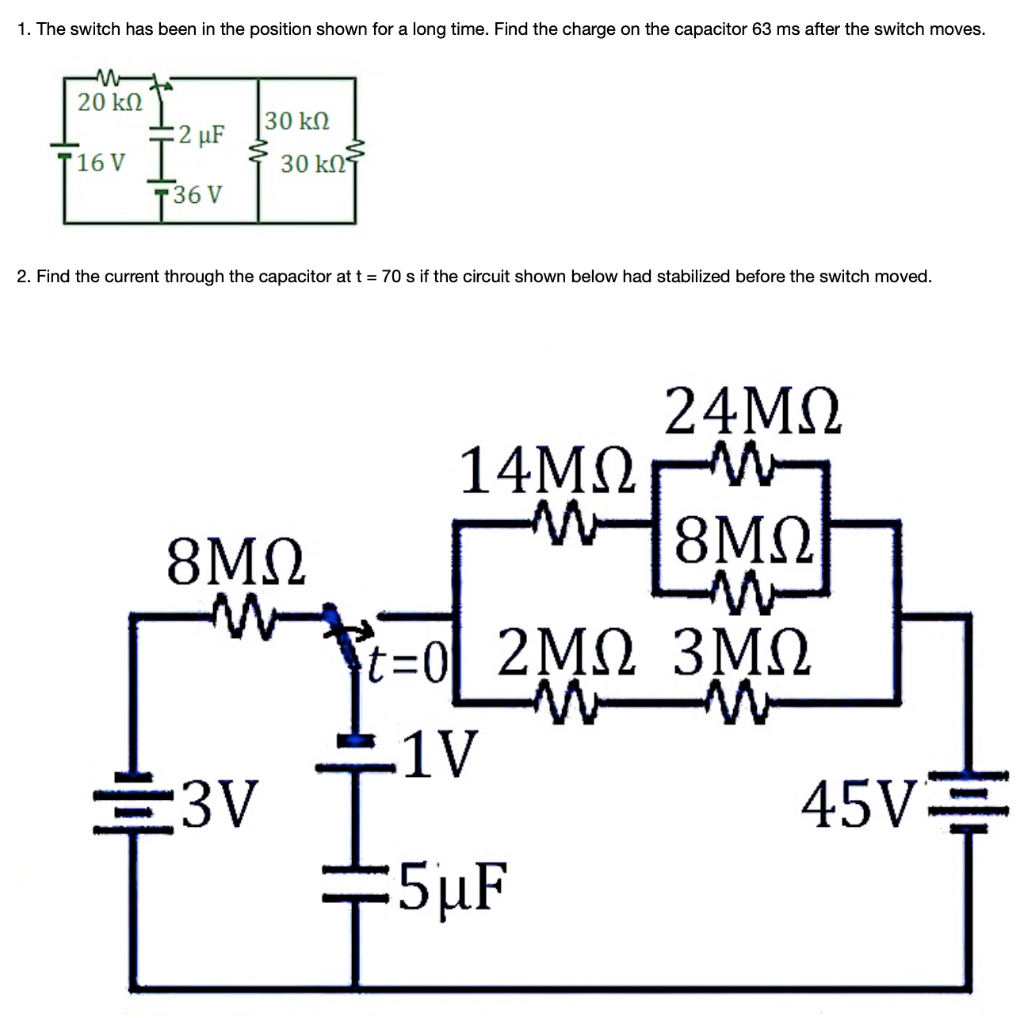1. The switch has been in the position shown for a long time. Find the charge on the capacitor 63 ms after the switch moves. 20 kΩ T16 V 30 kR T36 V 2. Find the current through the capacitor at t = 70 s if the circuit shown below had stabilized before the switch moved. 24ΜΩ 1V 45V

• ### Circuit 1. Assume that the switch has been in position 1 for a long time, and...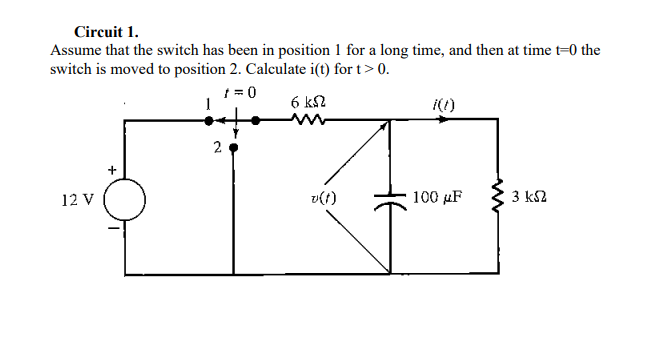Circuit 1. Assume that the switch has been in position 1 for a long time, and then at time t=0 the switch is moved to position 2. Calculate i(t) fort > 0. = 06k 2 12 V ve 100 uF 3 k2 Circuit 1: Find v(0), i.e. the capacitor voltage at time 0 (in Volts). 2.4 Circuit 1: What is v(t), approximately, for extremely larget, for example, for t= 10000000 s 30000 v 4V ο Ον

• ### 2. The switch in the circuit shown in Figure 1 has been in position 'a' for...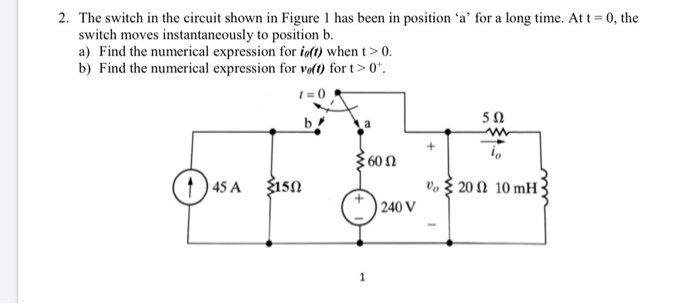2. The switch in the circuit shown in Figure 1 has been in position 'a' for a long time. At t=0, the switch moves instantaneously to position b. a) Find the numerical expression for io(t) when t > 0. b) Find the numerical expression for volt) fort > 0 1 = 0 b! 51 602 45 A 2150 vo 20 10 mH 240 V 1

• ### 7.55 The switch in the circuit of Fig. P 7.55 has been in position a for a long time. At t- 0 the switch is moved to position b. Calculate (a) the initial voltage on the capacitor; (b) the final volt...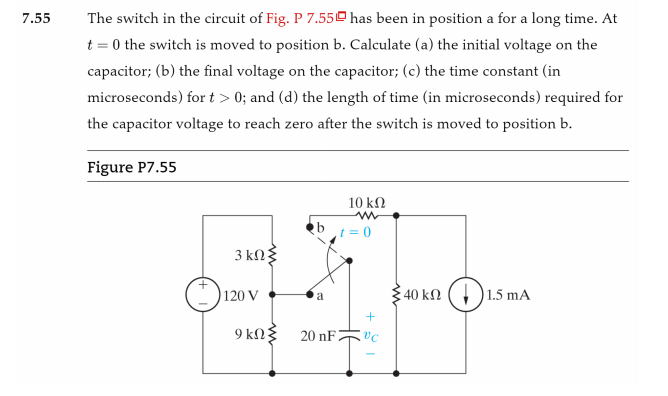7.55 The switch in the circuit of Fig. P 7.55 has been in position a for a long time. At t- 0 the switch is moved to position b. Calculate (a) the initial voltage on the capacitor; (b) the final voltage on the capacitor; (c) the time constant (in microseconds) for t 0; and (d) the length of time (in microseconds) required for the capacitor voltage to reach zero after the switch is moved to position b. Figure P7.55 10...

• ### Example1 The switch has been in position "a" for a long time. At time t-0, the switch moves from ...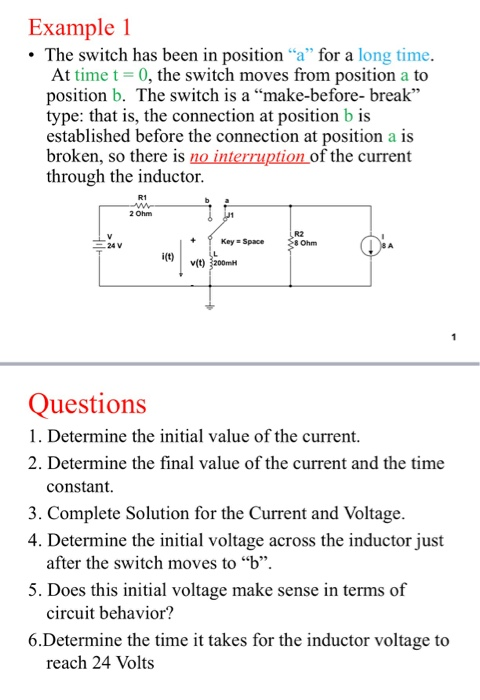Example1 The switch has been in position "a" for a long time. At time t-0, the switch moves from position a to position b. The switch is a "make-before- break" type: that is, the connection at position b is established before the connection at position a is broken, so there is no interruption of the current through the inductor R1 2 Ohm Key Space R2 8 Ohm 24 V i(t) v(t) Questions 1. Determine the initial value of the current....

• ### The switch in (Figure 1) has been in position a for a long time. It is...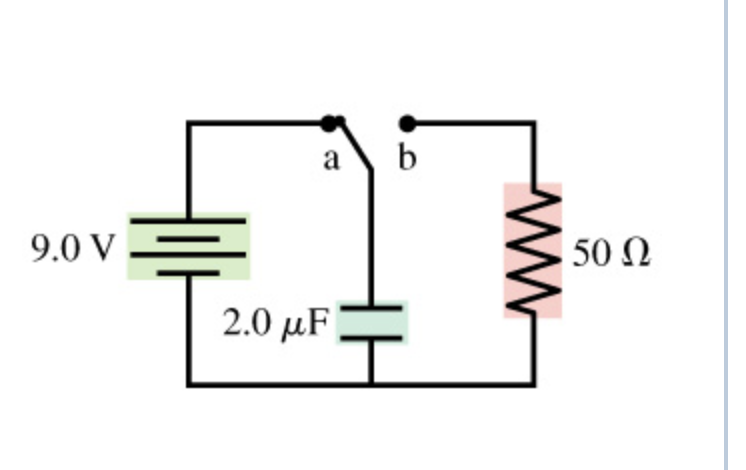The switch in (Figure 1) has been in position a for a long time. It is changed to position b at t = 0 s. a. What is the charge Q on the capacitor immediately after the switch is changed? Express your answer with the appropriate units. b. What is the current II through the resistor immediately after the switch is changed? Express your answer with the appropriate units. c. What is the charge Q on the capacitor at t...

• ### For the circuit in figure below, the switch has been in position a for a long...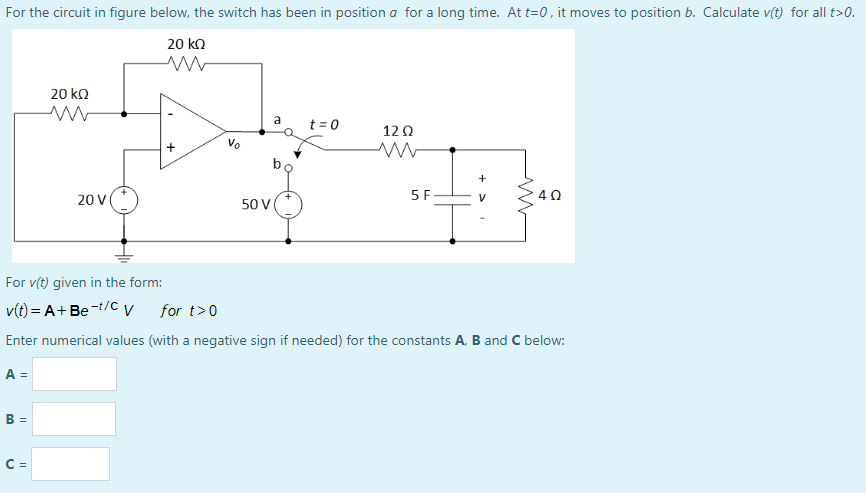For the circuit in figure below, the switch has been in position a for a long time. At t=0, it moves to position b. Calculate v(t) for all t>0. 20 ko 20 kΩ a t = 0 1202 + Vo + 20 V 5 F V 40 50 V For v(t) given in the form: v(t)=A+Be-t/c v for t> Enter numerical values (with a negative sign if needed) for the constants A, B and C below: A = B =...